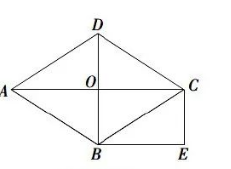(1) 求证: 四边形 $O C E B$ 是矩形.
(2) 连接 $D E$, 若 $\sin \angle C A B=\frac{3}{5}$, 则 $\tan \angle B D E$ 的值是【答案】 证明: (1) $\because$ 四边形 $A B C D$ 是菱形,
\begin{aligned} & \therefore A C \perp B D, \\ & \therefore \angle B O C=90^{\circ}, \\ & \because B E \perp B D, \\ & \therefore A C B E, \\ & \because B E=O C, \end{aligned}

$\therefore$ 四边形 $O C E B$ 是平行四边形,

$\therefore$ 平行四边形 $O C E B$ 是矩形;

(2) 解: $\frac{2}{3}$.